# How to Multiply in Microsoft Excel

In Microsoft Excel, you can solve mathematical equations using formulas. This spreadsheet processor offers basic mathematical functions that include addition, subtraction, multiplication, and division.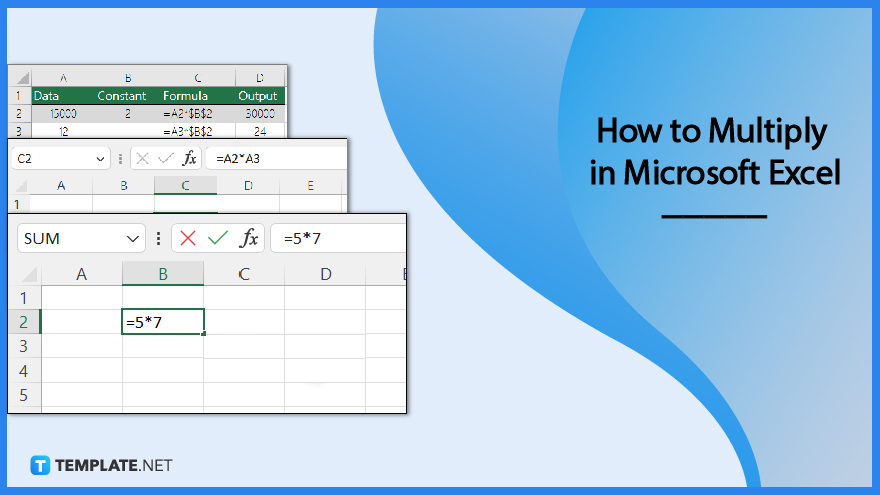## How to Multiply in Microsoft Excel

In Excel, numbers can be multiplied within a single cell, across multiple cells, or even across an entire column. You can also multiply numbers in different cells using a formula.

• ### Step 1: Multiply Numbers in a Cell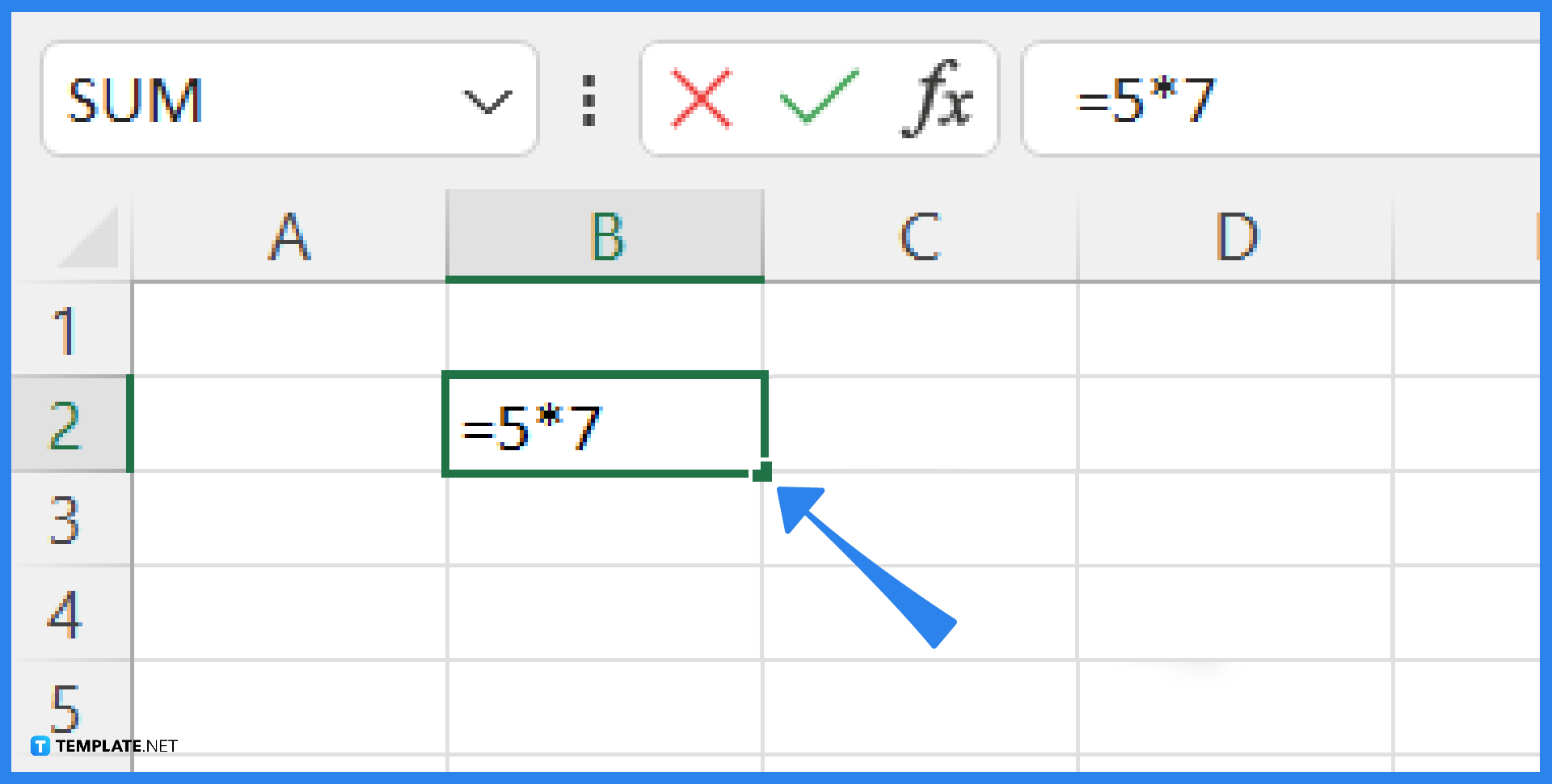A basic formula can be used to multiply numbers in a single cell. Open Excel, then pick the cell where you want to enter the equation. Once picked, type the formula: =a*b. Replace a and b with the numbers you’d like to multiply and press Enter to get the result.

• ### Step 2: Multiply Numbers From Multiple Cells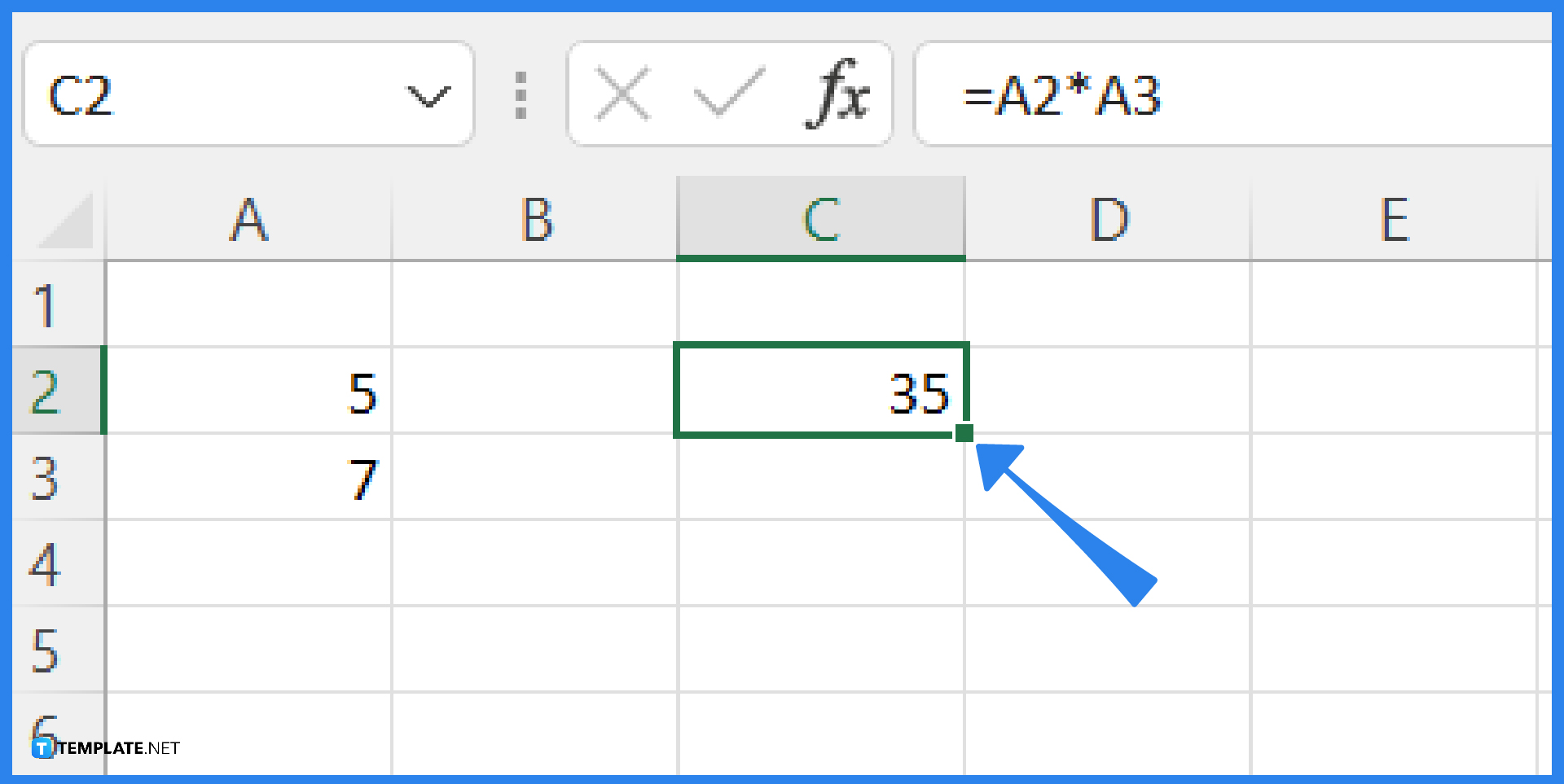To multiply data from other cells, enter a formula in a blank cell. Simply input the cell references. For example, “=A2*A3”. The formula pulls the data from each cell that has been entered for calculation. To get the result, press Enter.

• ### Step 3: Multiply a Column of Numbers by a Constant Number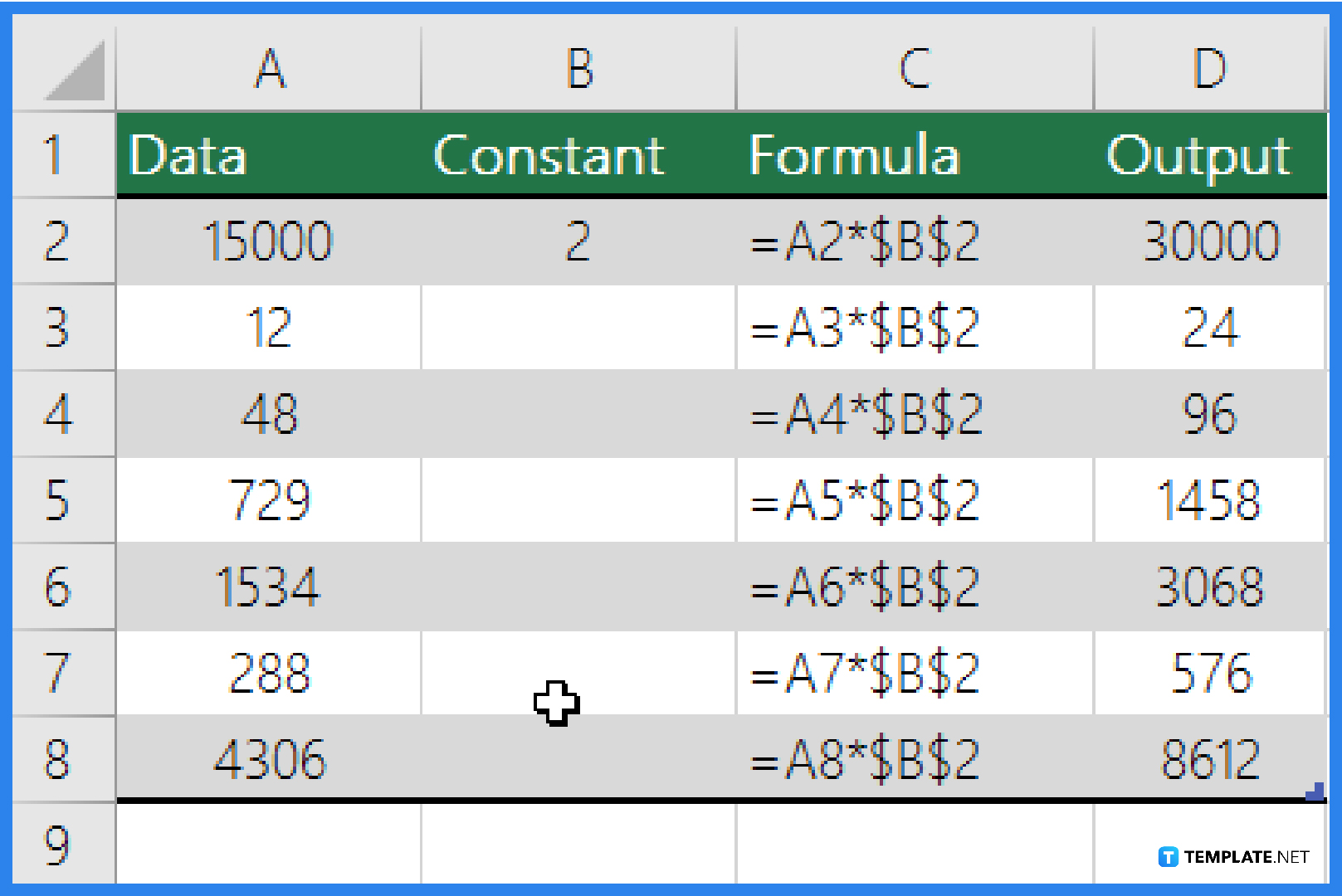You can multiply a column of numbers by a constant number in a different cell. Let’s say you want to multiply each of the seven numbers in a column by a value that is present in a different cell. For example, the number you want to multiply by is 2, contained in cell B2. First, type =A2*\$B\$2 in a new column in your spreadsheet. Make sure to place a \$ sign in the formula before B and before 2. Then, press Enter. After that, drag the formula down to the other cells in the column for their corresponding results.

•• ### Step 4: Multiply Numbers in Different Cells by Using a Formula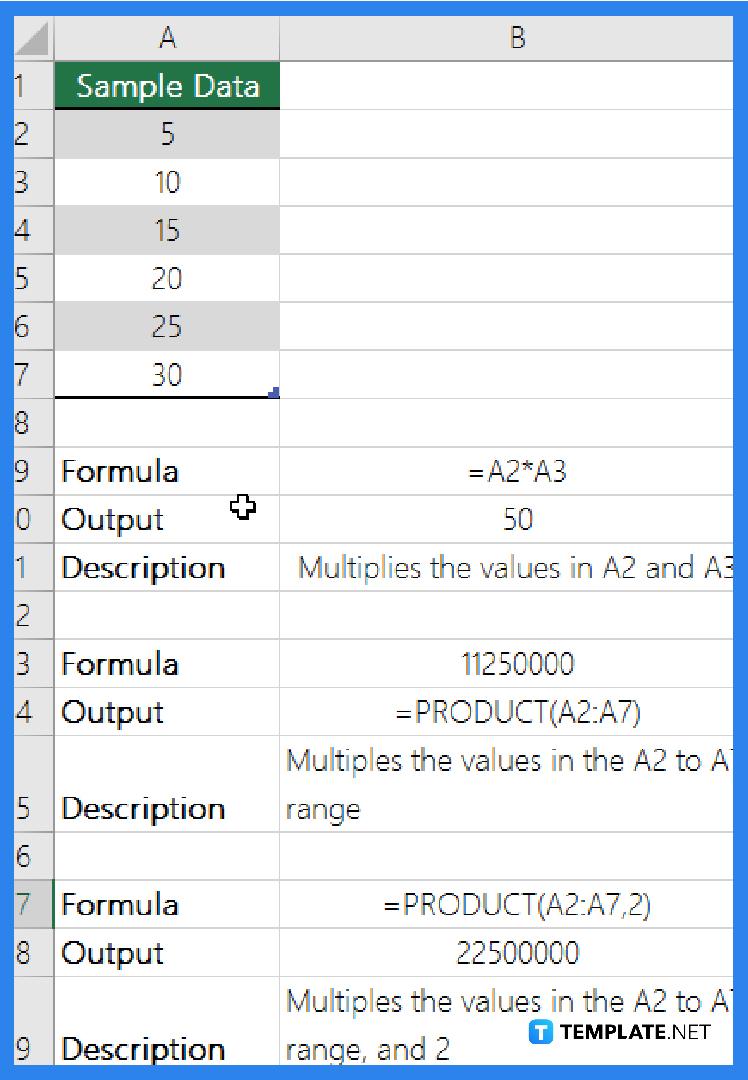To multiply numbers, cells, and ranges, use the PRODUCT function. In the PRODUCT function, you can use any combination of up to 255 numbers or cell references. For example, the formula =PRODUCT(A2,A4:A15,12,E3:E5,150,G4,H4:J6) multiplies two single cells (A2 and G4), two numbers (12 and 150), and three ranges (A4:A15, E3:E5, and H4:J6).

## FAQs

### Why should I learn how to multiply in Excel?

Working with datasets can be faster if you know how to multiply in Excel. Consider that you are working on a financial statement with a row that has decimal values. You can easily translate this row into percentages without using an electronic calculator if you know how to multiply using Microsoft Excel. Similarly, knowing how to multiply in Excel can aid in the computation of metrics like growth rates, standard deviations, attrition rates, net pay, and variable costs.

### How to multiply a number by a percentage in Excel?

Start by determining the quantity that you wish to increase or decrease by a certain percentage. After typing it in one cell, type the percentage sign in another cell. The next step is to write a formula for the multiplication operation you want to use. For example, you write the quantity in B2 and the percent in C2, so you write the formula as =B2*(1-C2).

### What Excel shortcuts can I use for multiplying numbers?

You can use the typical keys such as Ctrl + C, Ctrl + V, and Ctrl + A. When using the PRODUCT function, you can begin to type it and then press the “Tab” key to have Excel carry out the function for you. This method can also help you remember what the PRODUCT function does because Microsoft Excel contains function descriptions.